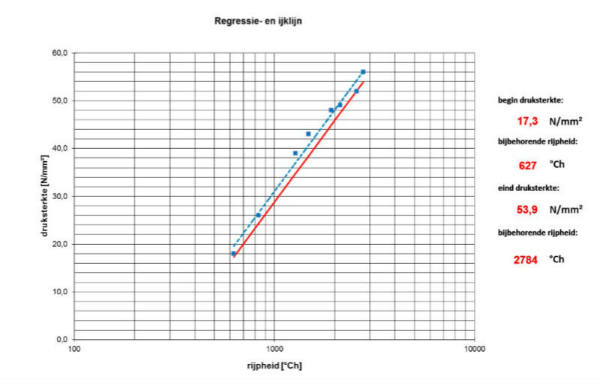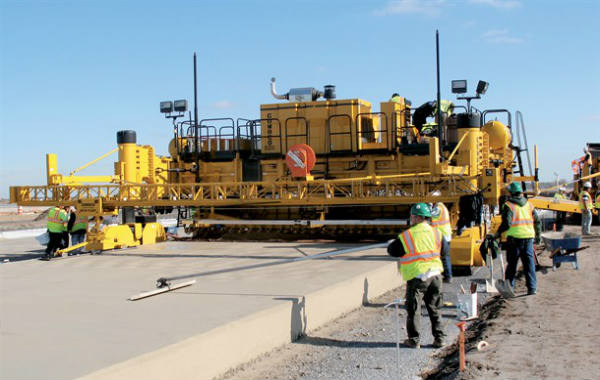﻿ Beton KalibrasyonuBETON OLGUNLUK MERKEZİ

## TANIMLARGRAPH PRINCIPLE:

• Make five cubes. Let these cubes cure at a temperature of about 20 °C. Determinate the compressive strength of these cubes on different times.
• Calculate the corresponding maturity of the cubes with the known C-value. Calculate and draw the regression line. Draw the calibration line.

PROCEDURE:

• Make the concrete mixture for which you want to determine the calibration graph. Fill five moulds of 150 mm x 150 mm x 150 mm with this mixture.
• Place the cubes including the moulds immediately after making in a thermostatic bath 20 ± 2°C. Record the time at the moment of placing. Take measures to prevent the leaching of material that has not yet cured.
• Prevent as far as possible the test specimens of drying out and cooling during and after stripping.
• Record the temperature in the heart of at least one cube. At least three temperature measurements per hour shall be carried out.
• Determine the compressive strength immediately after removing from the water.
• Determine the compressive strength of the cubes at such times that a range of a maximum of 8 N/mm2 below to a maximum of 8 N/mm2 above the intended strength is covered. Example: If on the building site a compressive strength of 12 N/mm²–16 N/mm² is required, we take 14 N/mm² as the intended compressive strength. The range will be then from 6 N/mm² to 22 N/mm².
• Make sure that the observations are equally divided over the area, where there shall be at least two measured values below and two measured values above the intented strength.
• Calculate the weighted maturity for every cube on the time of pressing, from the hardening time and concrete temperature.
• Plot the results in a graph against the compressive strength. On the x-axis, on a logarithmic scale, the maturity and on the y-axis, on a linear scale, the compressive strength shall be shown.
• Calculate and draw the regression line. Extrapolation outside the measurement area is not allowed.

EQUIPMENT AND DEVICES :

• A thermostatic bath 20 ± 2 °C
• Thermocouple wires, with corresponding recording equipment.

CHECK :

• A regular check of a calibration graph shall be carried out at least once every two weeks using at least one concrete cube.
• If the period between two production batches is longer than two weeks the check shall only be carried out upon delivery.
• If the period between two production batches is longer than three months, a new calibration graph shall be drawn up.
• If the measured compressive strength of the control cube is under the calibration line, a second check shall be carried out. If the compressive strength of the second control cube is again under the calibration line, a new regression line shall be calculated.
• It is possible to draw up a completely new calibration graph at any time.

REPORT : A report must contain all important information. It must be clear for which concrete mixture the calibration graph is and what is de C-value of the used binding agent.

Betonun Kalibrasyonunun Belirlenmesi (TS 13508) dokümanını indirmek için tıklayınız.